# 1 IAEA 2D PWR Benchmark

#                       BENCHMARK PROBLEM
#
# Identification: 11-A2          Source Situation ID.11
# Date Submitted: June 1976      By: R. R. Lee (CE)
#                                    D. A. Menely (Ontario Hydro)
#                                    B. Micheelsen (Riso-Denmark)
#                                    D. R. Vondy (ORNL)
#                                    M. R. Wagner (KWU)
#                                    W. Werner (GRS-Munich)
#
# Date Accepted:  June 1977      By: H. L. Dodds, Jr. (U. of Tenn.)
#                                    M. V. Gregory (SRL)
#
# Descriptive Title: Two-dimensional LWR Problem,
#                    also 2D IAEA Benchmark Problem
#
# Reduction of Source Situation
#           1. Two-groupo diffusion theory
#           2. Two-dimensional (x,y)-geometry
#
PROBLEM neutron_diffusion 2D GROUPS 2
DEFAULT_ARGUMENT_VALUE 1 quarter   # either quarter or eigth
READ_MESH iaea-2dpwr-$1.msh # define materials and cross sections according to the two-group constants # each material corresponds to a physical entity in the geometry file Bg2 = 0.8e-4 # axial geometric buckling in the z direction MATERIAL fuel1 { D1=1.5 Sigma_a1=0.010+D1(x,y)*Bg2 Sigma_s1.2=0.02 D2=0.4 Sigma_a2=0.080+D2(x,y)*Bg2 nuSigma_f2=0.135 }#eSigmaF_2 nuSigmaF_2(x,y) } MATERIAL fuel2 { D1=1.5 Sigma_a1=0.010+D1(x,y)*Bg2 Sigma_s1.2=0.02 D2=0.4 Sigma_a2=0.085+D2(x,y)*Bg2 nuSigma_f2=0.135 }#eSigmaF_2 nuSigmaF_2(x,y) } MATERIAL fuel2rod { D1=1.5 Sigma_a1=0.010+D1(x,y)*Bg2 Sigma_s1.2=0.02 D2=0.4 Sigma_a2=0.130+D2(x,y)*Bg2 nuSigma_f2=0.135 }#eSigmaF_2 nuSigmaF_2(x,y) } MATERIAL reflector { D1=2.0 Sigma_a1=0.000+D1(x,y)*Bg2 Sigma_s1.2=0.04 D2=0.3 Sigma_a2=0.010+D2(x,y)*Bg2 } # define boundary conditions as requested by the problem BC external vacuum=0.4692 # "external" is the name of the entity in the .geo BC mirror mirror # the first mirror is the name, the second is the BC type # # set the power setpoint equal to the volume of the core # # (and set eSigmaF_2 = nuSigmaF_2 as above) # power = 17700 SOLVE_PROBLEM # solve! PRINT %.5f "keff = " keff WRITE_MESH iaea-2dpwr-$1.vtk phi1 phi2
$gmsh -2 iaea-2dpwr-quarter.geo$ [...]
$gmsh -2 iaea-2dpwr-eighth.geo$ [...]
$feenox iaea-2dpwr.fee quarter keff = 1.02986$ feenox iaea-2dpwr.fee eighth
keff =  1.02975
$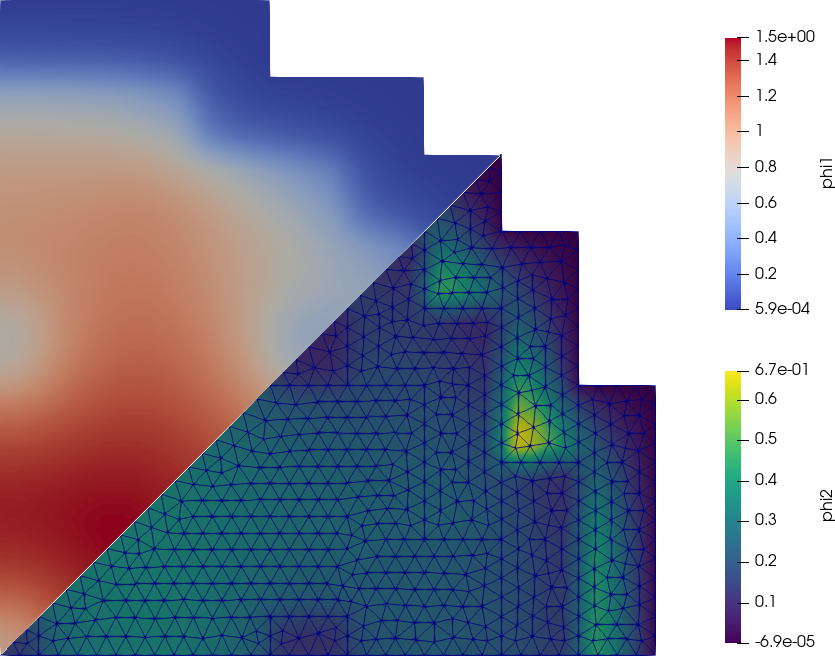Fast and thermal flux for the 2D IAEA PWR benchmark # 2 IAEA 3D PWR Benchmark The IAEA 3D PWR Benchmark is a classical problem for core-level diffusion codes. The original geometry, cross sections and boundary conditions are shown in figs. 1-3.Figure 1: The IAEA 3D PWR Benchmark, fig. 1Figure 2: The IAEA 3D PWR Benchmark, fig. 2Figure 3: The IAEA 3D PWR Benchmark, fig. 3 The FeenoX approach consists of modeling both the original one-quarter-symmetric geometry and the more reasonable one-eighth-symmetry geometry in a 3D CAD cloud tool such as Onshape (figs. 4, 5). Then, the CAD is imported and meshed in Gmsh to obtain a second-order unstructured tetrahedral grids suitable to be used by FeenoX to solve the multi-group neutron diffusion equation (figs. 6, 7)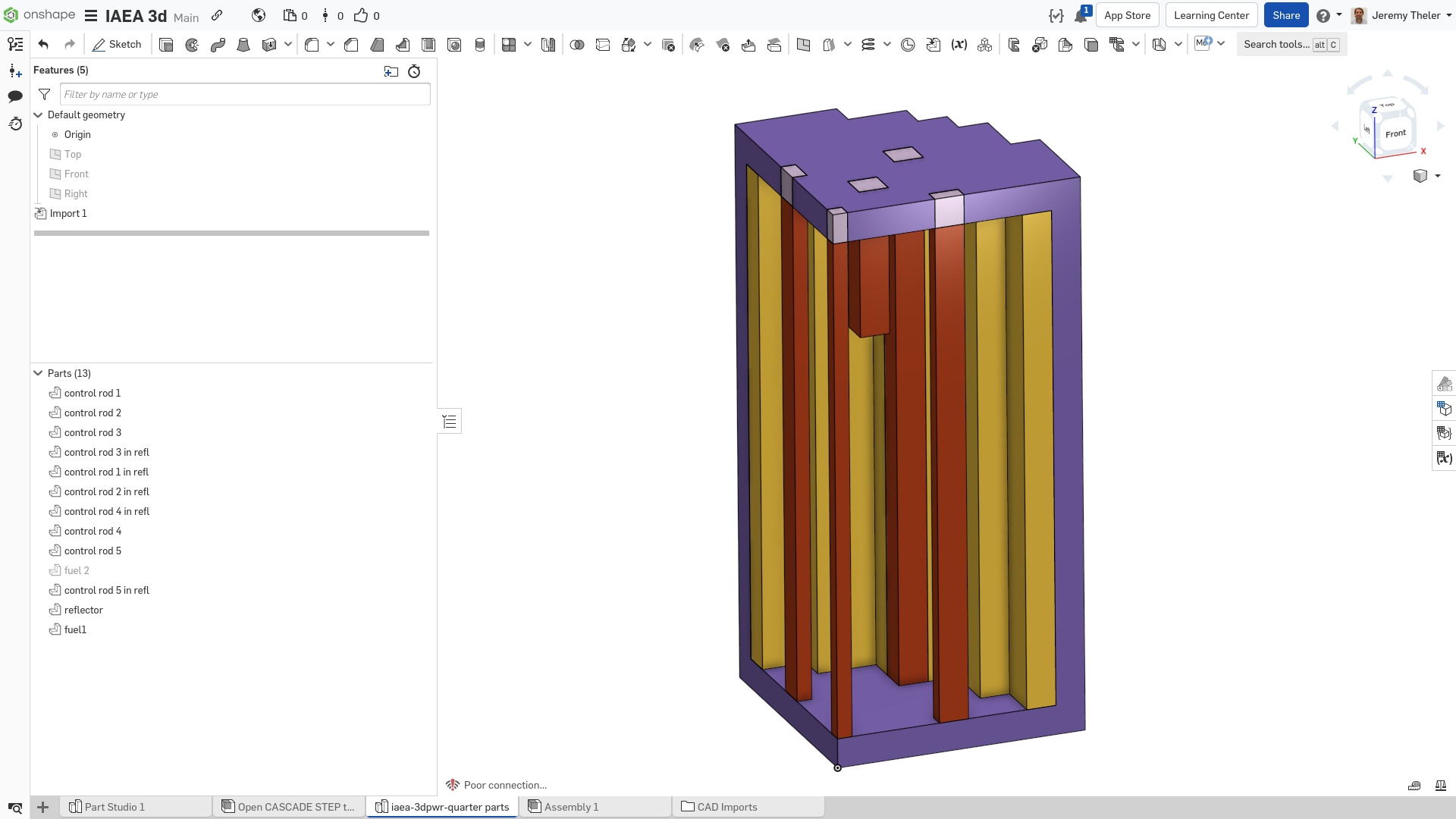Figure 4: IAEA 3D PWR one-quarter CAD in Onshape (“fuel 2” is hidden)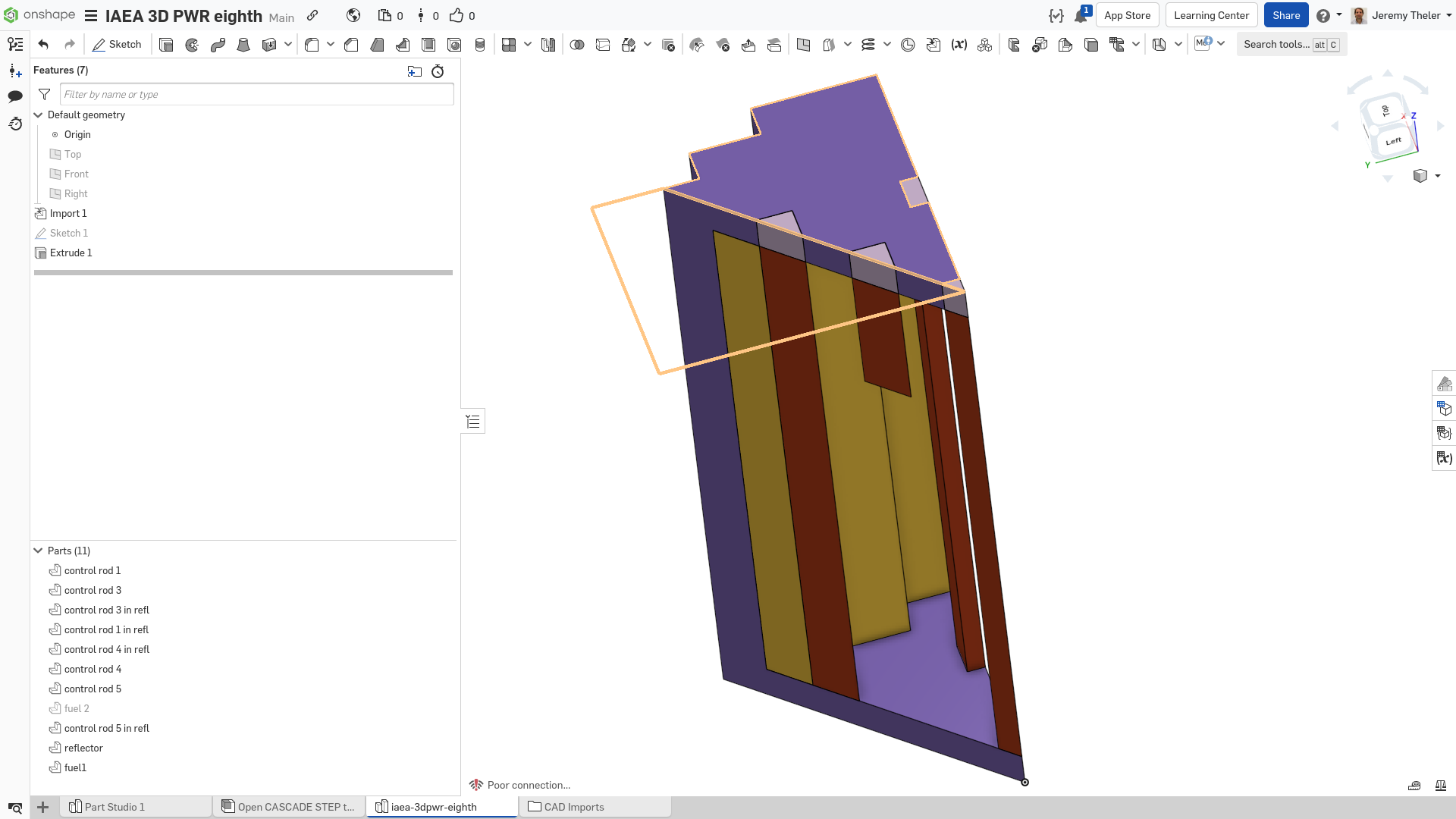Figure 5: IAEA 3D PWR one-eighth CAD in Onshape (“fuel 2” is hidden)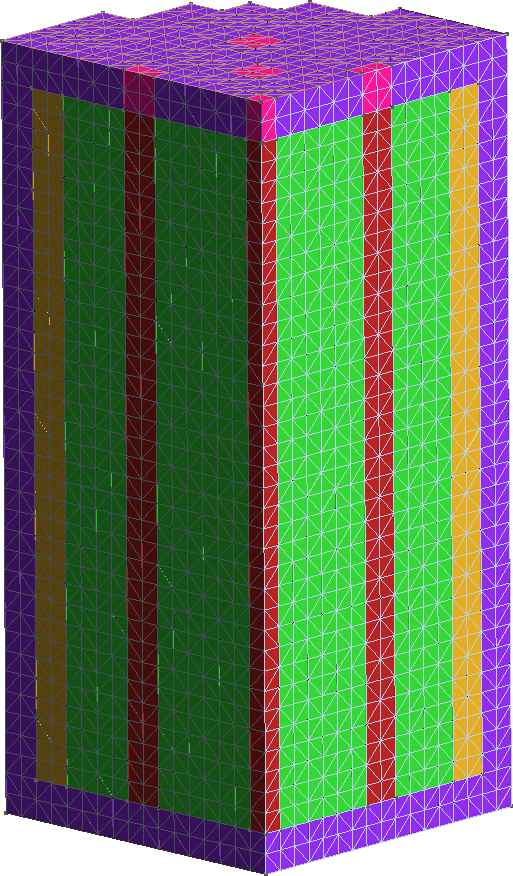Figure 6: Unstructured second-order tetrahedral grid for the quarter case. a — Full view, b — Cutaway view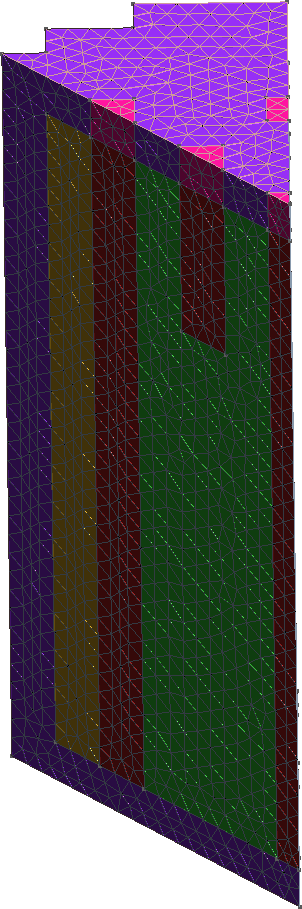Figure 7: Unstructured second-order tetrahedral grid for the eighth case. a — Full view, b — Cutaway view The terminal mimic shows that the eighth case can be solved faster and needs less memory than the original quarter-symmetry case. Recall that the original problem does have 1/8th symmetry but since historically all core-level solvers can only handle structured hexahedral grids, nobody ever took advantage of it. # BENCHMARK PROBLEM # # Identification: 11 # Date Submitted: June 1976 By: R. R. Lee (CE) # D. A. Menely (Ontario Hydro) # B. Micheelsen (Riso-Denmark) # D. R. Vondy (ORNL) # M. R. Wagner (KWU) # W. Werner (GRS-Munich) # # Date Accepted: June 1977 By: H. L. Dodds, Jr. (U. of Tenn.) # M. V. Gregory (SRL) # # Descriptive Title: Multi-dimensional (x-y-z) LWR model # # Suggested Functions: Designed to provide a sever test for # the capabilities of coarse mesh # methods and flux synthesis approximations # # Configuration: Three-dimensional configuration # including space dimensions and region # numbers: 2 Figures t0 = clock() # start measuring wall time PROBLEM neutron_diffusion 3D GROUPS 2 DEFAULT_ARGUMENT_VALUE 1 quarter READ_MESH iaea-3dpwr-$1.msh

MATERIAL fuel1     D1=1.5 D2=0.4 Sigma_s1.2=0.02 Sigma_a1=0.01 Sigma_a2=0.08  nuSigma_f2=0.135
MATERIAL fuel2     D1=1.5 D2=0.4 Sigma_s1.2=0.02 Sigma_a1=0.01 Sigma_a2=0.085 nuSigma_f2=0.135
MATERIAL fuel2rod  D1=1.5 D2=0.4 Sigma_s1.2=0.02 Sigma_a1=0.01 Sigma_a2=0.13  nuSigma_f2=0.135
MATERIAL reflector D1=2.0 D2=0.3 Sigma_s1.2=0.04 Sigma_a1=0    Sigma_a2=0.01  nuSigma_f2=0
MATERIAL reflrod   D1=2.0 D2=0.3 Sigma_s1.2=0.04 Sigma_a1=0    Sigma_a2=0.055 nuSigma_f2=0

BC vacuum   vacuum=0.4692
BC mirror   mirror

SOLVE_PROBLEM
WRITE_RESULTS FORMAT vtk

# print results
PRINT SEP " " "  keff = " %.5f keff
PRINT SEP " " " nodes = " %g   nodes
PRINT SEP " " "memory = " %.1f memory() "Gb"
PRINT SEP " " "  wall = " %.1f clock()-t0 "sec"
$gmsh -3 iaea-3dpwr-quarter.geo$ [...]
$gmsh -3 iaea-3dpwr-eighth.geo$ [...]
$feenox iaea-3dpwr.fee quarter keff = 1.02918 nodes = 70779 memory = 3.9 Gb wall = 33.8 sec$ feenox iaea-3dpwr.fee eighth
keff =  1.02912
nodes =  47798
memory =  2.5 Gb
wall =  16.0 sec
$Figure 8: Fast flux for the 3D IAEA PWR benchmark in 1/8th symmetry.Figure 9: Thermal flux for the 3D IAEA PWR benchmark in 1/8th symmetry. # 3 Cube-spherical bare reactor It is easy to compute the effective multiplication factor of a one-group bare cubical reactor. Or a spherical reactor. And we know that for the same mass, the k_\text{eff} for the former is smaller than for the latter.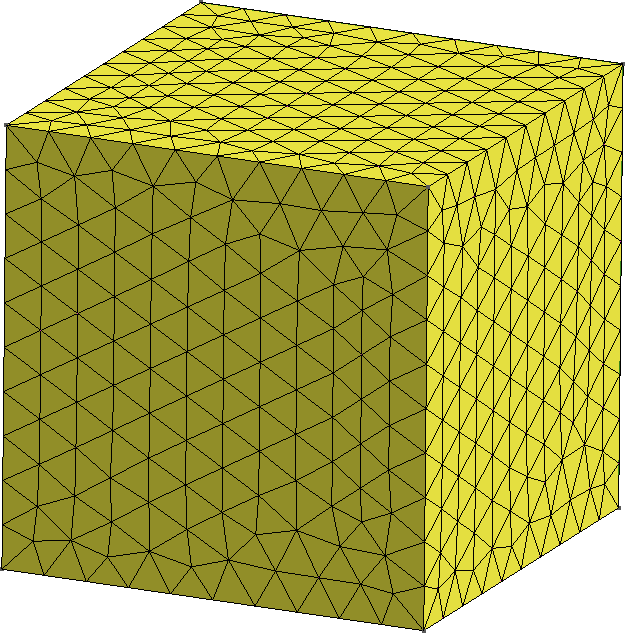Figure 10: One eight of two bare reactors. a — Cubical reactor, b — Spherical reactor But what happens “in the middle”? That is to say, how does k_\text{eff} changes when we morph the cube into a sphere? Enter Gmsh & Feenox.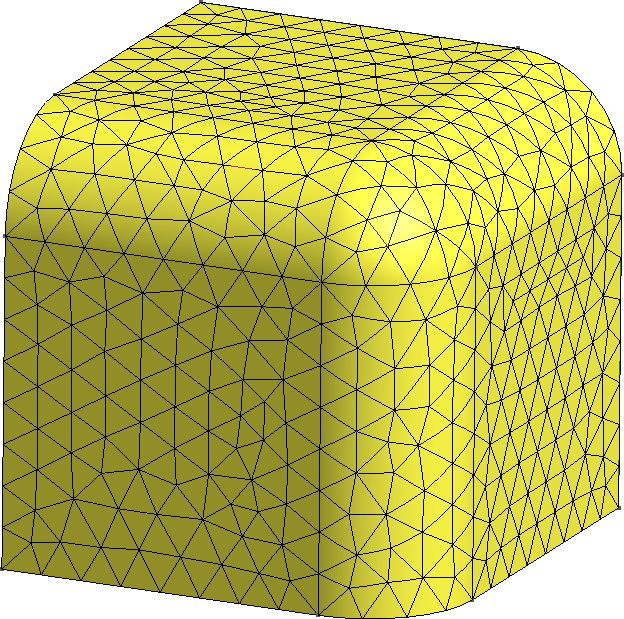Figure 11: Continuous morph between a cube and a sphere. a — 75% cube/25% sphere, b — 50% cube/50% sphere, c — 25% cube/75% sphere import os import math import gmsh def create_mesh(vol, F): gmsh.initialize() gmsh.option.setNumber("General.Terminal", 0) f = 0.01*F a = (vol / (1/8*4/3*math.pi*f**3 + 3*1/4*math.pi*f**2*(1-f) + 3*f*(1-f)**2 + (1-f)**3))**(1.0/3.0) internal = [] gmsh.model.add("cubesphere") if (F < 1): # a cube gmsh.model.occ.addBox(0, 0, 0, a, a, a, 1) internal = [1,3,5] external = [2,4,6] elif (F > 99): # a sphere gmsh.model.occ.addSphere(0, 0, 0, a, 1, 0, math.pi/2, math.pi/2) internal = [2,3,4] external =  else: gmsh.model.occ.addBox(0, 0, 0, a, a, a, 1) gmsh.model.occ.fillet(, [12, 7, 6], [f*a], True) internal = [1,4,6] external = [2,3,5,7,8,9,10] gmsh.model.occ.synchronize() gmsh.model.addPhysicalGroup(3, , 1) gmsh.model.setPhysicalName(3, 1, "fuel") gmsh.model.addPhysicalGroup(2, internal, 2) gmsh.model.setPhysicalName(2, 2, "internal") gmsh.model.addPhysicalGroup(2, external, 3) gmsh.model.setPhysicalName(2, 3, "external") gmsh.model.occ.synchronize() gmsh.option.setNumber("Mesh.ElementOrder", 2) gmsh.option.setNumber("Mesh.Optimize", 1) gmsh.option.setNumber("Mesh.OptimizeNetgen", 1) gmsh.option.setNumber("Mesh.HighOrderOptimize", 1) gmsh.option.setNumber("Mesh.CharacteristicLengthMin", a/10); gmsh.option.setNumber("Mesh.CharacteristicLengthMax", a/10); gmsh.model.mesh.generate(3) gmsh.write("cubesphere-%g.msh"%(F)) gmsh.model.remove() #gmsh.fltk.run() gmsh.finalize() return def main(): vol0 = 100**3 for F in range(0,101,5): # mesh refinement level create_mesh(vol0, F) # TODO: FeenoX Python API! os.system("feenox cubesphere.fee %g"%(F)) if __name__ == "__main__": main() PROBLEM neutron_diffusion DIMENSIONS 3 READ_MESH cubesphere-$1.msh DIMENSIONS 3

# MATERIAL fuel
D1 = 1.03453E+00
Sigma_a1 = 5.59352E-03
nuSigma_f1 = 6.68462E-03
Sigma_s1.1 = 3.94389E-01

PHYSICAL_GROUP fuel DIM 3
BC internal    mirror
BC external    vacuum

SOLVE_PROBLEM

PRINT HEADER $1 keff 1e5*(keff-1)/keff fuel_volume $ python cubesphere.py | tee cubesphere.dat
0       1.05626 5326.13 1e+06
5       1.05638 5337.54 999980
10      1.05675 5370.58 999980
15      1.05734 5423.19 999992
20      1.05812 5492.93 999995
25      1.05906 5576.95 999995
30      1.06013 5672.15 999996
35      1.06129 5775.31 999997
40      1.06251 5883.41 999998
45      1.06376 5993.39 999998
50      1.06499 6102.55 999998
55      1.06619 6208.37 999998
60      1.06733 6308.65 999998
65      1.06839 6401.41 999999
70      1.06935 6485.03 999998
75      1.07018 6557.96 999998
80      1.07088 6618.95 999998
85      1.07143 6666.98 999999
90      1.07183 6701.24 999999
95      1.07206 6721.33 999998
100     1.07213 6727.64 999999
$Static reactivity vs. percentage of sphericity # 4 Illustration of the XS dilution & smearing effect The best way to solve a problem is to avoid it in the first place. Richard M. Stallman Let us consider a two-zone slab reactor: 1. Zone A has k_\infty < 1 and extends from x=0 to x=a. 2. Zone B has k_\infty > 1 and extends from x=a to x=b. • The slab is solved with a one-group diffusion approach. • Both zones have uniform macroscopic cross sections. • Flux \phi is equal to zero at both at x=0 and at x=b. Under these conditions, the overall analytical effective multiplication factor is k_\text{eff} such that \sqrt{D_A\cdot\left(\Sigma_{aA}- \frac{\nu\Sigma_{fA}}{k_\text{eff}}\right)} \cdot \tan\left[\sqrt{\frac{1}{D_B} \cdot\left( \frac{\nu\Sigma_{fB}}{k_\text{eff}}-\Sigma_{aB} \right) }\cdot (a-b) \right] = \sqrt{D_B\cdot\left(\frac{\nu\Sigma_{fB}}{k_\text{eff}}-\Sigma_{aB}\right)} \cdot \tanh\left[\sqrt{\frac{1}{D_A} \cdot\left( \Sigma_{aA}-\frac{\nu\Sigma_{fA}}{k_\text{eff}} \right)} \cdot b\right] We can then compare the numerical k_\text{eff} computed using… 1. a non-uniform grid with n+1 nodes such that there is a node exactly at x=b. 2. an uniform grid (mimicking a neutronic code that cannot handle case i.) with n uniformly-spaced elements. The element that contains point x=b is assigned to a pseudo material AB that linearly interpolates the macroscopic cross sections according to where in the element the point x=b lies. That is to say, if the element width is 10 and b=52 then this AB material will be 20% of material A and 80% of material B. The objective of this example is to show that case i. will monotonically converge to the analytical multiplication factor as n \rightarrow \infty while case ii. will show a XS dilution and smearing effect. FeenoX of course can solve both cases, but there are many other neutronic tools out there that can handle ony structured grids. #!/bin/bash b="100" # total width of the slab if [ -z$1 ]; then
n="10"    # number of cells
else
n=$1 fi rm -rf two-zone-slab-*-${n}.dat

# sweep a (width of first material) between 10 and 90
for a in $(seq 35 57); do cat << EOF > ab.geo a =${a};
b = ${b}; n =${n};
lc = b/n;
EOF
for m in uniform nonuniform; do
gmsh -1 -v 0 two-zone-slab-${m}.geo feenox two-zone-slab.fee${m} | tee -a two-zone-slab-${m}-${n}.dat
done
done
PROBLEM neutron_diffusion 1D
DEFAULT_ARGUMENT_VALUE 1 nonuniform
READ_MESH two-zone-slab-$1.msh # this ab.geo is created from the driving shell script INCLUDE ab.geo # pure material A from x=0 to x=a D1_A = 0.5 Sigma_a1_A = 0.014 nuSigma_f1_A = 0.010 # pure material B from x=a to x=b D1_B = 1.2 Sigma_a1_B = 0.010 nuSigma_f1_B = 0.014 # meta-material (only used for uniform grid to illustrate XS dilution) a_left = floor(a/lc)*lc; xi = (a - a_left)/lc Sigma_tr_A = 1/(3*D1_A) Sigma_tr_B = 1/(3*D1_B) Sigma_tr_AB = xi*Sigma_tr_A + (1-xi)*Sigma_tr_B D1_AB = 1/(3*Sigma_tr_AB) Sigma_a1_AB = xi * Sigma_a1_A + (1-xi)*Sigma_a1_B nuSigma_f1_AB = xi * nuSigma_f1_A + (1-xi)*nuSigma_f1_B BC left null BC right null SOLVE_PROBLEM # compute the analytical keff F1(k) = sqrt(D1_A*(Sigma_a1_A-nuSigma_f1_A/k)) * tan(sqrt((1/D1_B)*(nuSigma_f1_B/k-Sigma_a1_B))*(a-b)) F2(k) = sqrt(D1_B*(nuSigma_f1_B/k-Sigma_a1_B)) * tanh(sqrt((1/D1_A)*(Sigma_a1_A-nuSigma_f1_A/k))*b) k = root(F1(k)-F2(k), k, 1, 1.2) # # and the fluxes (not needed here but for reference) # B_A = sqrt((Sigma_a1_A - nuSigma_f1_A/k)/D1_A) # fluxA(x) = sinh(B_A*x) # # B_B = sqrt((nuSigma_f1_B/k - Sigma_a1_B)/D1_B) # fluxB(x)= sinh(B_A*b)/sin(B_B*(a-b)) * sin(B_B*(a-x)) # # # normalization factor # f = a/(integral(fluxA(x), x, 0, b) + integral(fluxB(x), x, b, a)) # flux(x) := f * if(x < b, fluxA(x), fluxB(x)) PRINT a keff k keff-k b n lc nodes # PRINT_FUNCTION flux MIN 0 MAX a STEP a/1000 FILE_PATH two-zone-analytical.dat # PRINT_FUNCTION phi1 phi1(x)-flux(x) FILE_PATH two-zone-numerical.dat $ ./two-zone-slab.sh 10
[...]
$./two-zone-slab.sh 20 [...]$ pyxplot two-zone-slab.ppl
\$